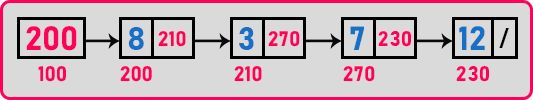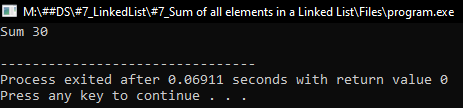# Sum of all Elements in a Linked List

## Sum of all Elements in a Linked List using C Language with Examples:

In this article, I am going to discuss How to find the Sum of all elements in a Linked List using C Language with Examples. Please read our previous article, where we discussed Counting Nodes in a Linked List using C Language with Examples.

##### How to find the Sum of all elements in a Linked list?

Here, we will write a function for finding the sum of all these elements in a linked list.We want to add all these elements one by one. So, for adding we have to traverse all the nodes. We need a pointer for traversing the nodes. So let us write a function for adding all these values.

###### Iterative Version:
```int Add(struct Node *p){
int sum = 0;
while(p){
sum += p->data;
p = p->next;
}
return(sum);
}
```

Now let us write the same function for finding the sum of all the elements using recursion.

###### Recursive Version:
```int Add(struct Node *p){
if(p == 0)
return 0;
else
}
```

The same recursive method we have already seen in the previous article where we were calculating the number of nodes in a linked list. Now let us write the full program in the C language.

##### Program to find the Sum of all the Elements in a Linked List using C Language:
```#include <stdio.h>
#include <stdlib.h>
struct Node
{
int data;
struct Node *next;
}   *first = NULL;

void Create(int A[], int n)
{
int i;
struct Node *t, *last;
first = (struct Node *) malloc (sizeof (struct Node));
first->data = A;
first->next = NULL;
last = first;

for (i = 1; i < n; i++)
{
t = (struct Node *) malloc (sizeof (struct Node));
t->data = A[i];
t->next = NULL;
last->next = t;
last = t;
}
}

{
int sum = 0;
while (p)
{
sum += p->data;
p = p->next;
}
return (sum);
}

{
if (p == 0)
return 0;
else
}

int main()
{
int A[] = { 8, 3, 7, 12 };
Create (A, 4);